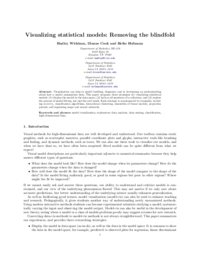# Visualizing statistical models: Removing the blindfold• Visualizing statistical models: Removing the blindfold.
• Statistical Analysis and Data Mining: The ASA Data Science Journal, vol. 8, no. 4, pp. 203–225, 2015.

Visualization can help in model building, diagnosis, and in developing an understanding about how a model summarizes data. This paper proposes three strategies for visualizing statistical models: (i) display the model in the data space, (ii) look at all members of a collection, and (iii) explore the process of model fitting, not just the end result. Each strategy is accompanied by examples, including manova, classification algorithms, hierarchical clustering, ensembles of linear models, projection pursuit, self-organizing maps, and neural networks.

```@Article{model-vis,
author = {Hadley Wickham and Dianne Cook and Heike Hofmann},
journal = {Statistical Analysis and Data Mining: The ASA Data Science Journal},
number = {4},
pages = {203–225},
title = {Visualizing statistical models: Removing the blindfold},
volume = {8},
year = {2015},
url = {http://onlinelibrary.wiley.com/doi/10.1002/sam.11271/full},
}```